# Multiplying Decimals by Whole Numbers

##By SmithMathAcademy

Multiplying Decimals by Whole Numbers# Multiplying decimals 2

##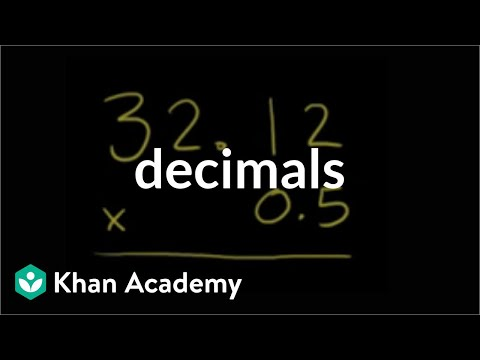By Khan Academy

Multiplying decimals? Try multiplying without the decimals first, them add them back in. We'll show you.# Multiplying decimals 3

##By Khan Academy

Multiplying decimals? Try multiplying without the decimals first, them add them back in. We'll show you.# Multiplying decimals 2

##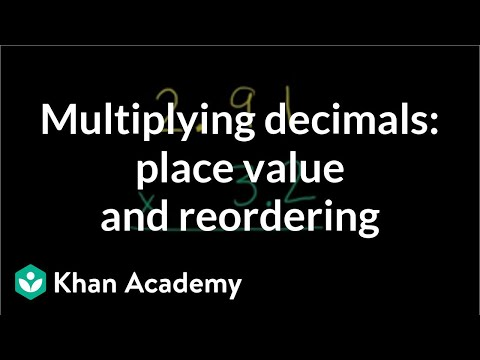By Khan Academy

We want you to develop an intuition about how to work with decimals. Understanding how you can rewrite decimals by considering the place value will help you multiply!# Multiplying decimals 1

##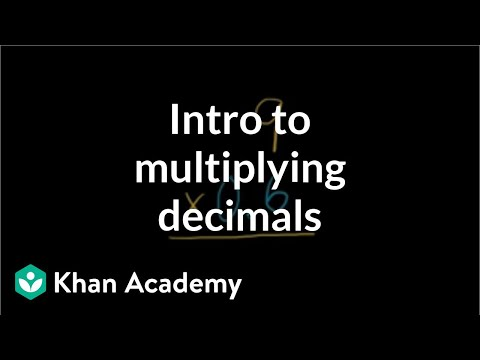By Khan Academy

Multiplying decimals can be confusing because there's always the question as to where the decimals goes in the answer. We're about to show you so you'll never have to question it again!# Multiplying decimals 1

##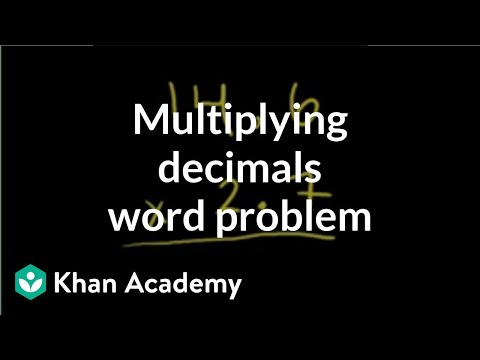By Khan Academy

We're multiplying decimals again, but this time answering a common question: what's the cost of filling up the car tank?# Multiplying and Dividing Decimals | 7.NS.A.2c | 7th Grade Math

##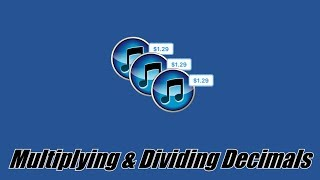By ParksMath | Todd Parks

ParksMath explains how to multiply and divide decimals numbers without the use of a calculator. Understanding how to change a decimal number so that it is an integer, makes multiplying and dividing decimals much easier. This quick tutorial will give you thee tools that you need to find the product or quotient of any decimal number.# Multiplying decimals 2

##By Khan Academy

Sometimes multiplying really small decimals (with all those zeros!) can be a little intimidating. Watch as we show you a handy trick to simplify these problems and solve them.# Multiplying decimals 3

##By Khan Academy

Sometimes multiplying really small decimals (with all those zeros!) can be a little intimidating. Watch as we show you a handy trick to simplify these problems and solve them.# More involved multiplying decimals example

##By Khan Academy

Sometimes multiplying really small decimals (with all those zeros!) can be a little intimidating. Watch as we show you a handy trick to simplify these problems and solve them.# Multiplying Decimals - On a Place Value Chart (5.NBT.B.7)

##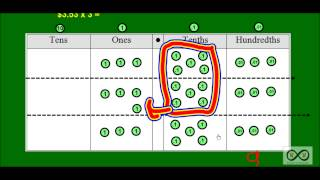By Worksheets and Walkthroughs

This video walkthrough lesson with corresponding worksheets investigates what happens on a place value chart as we multiply a decimal by a single digit number in a story problem format. Place value disks are used to represent values on a place value chart.# Multiplying and Dividing Decimals | 7.NS.A.2c | 7th Grade Math

##By ParksMath

ParksMath explains how to multiply and divide decimals numbers without the use of a calculator. Understanding how to change a decimal number so that it is an integer, makes multiplying and dividing decimals much easier. This quick tutorial will give you thee tools that you need to find the product or quotient of any decimal number.# Grade 5 Math - Multiply Decimals 1

##By Lumos Learning

Using the Lumos Study Programs, parents and educators can reinforce the classroom learning experience for children and help them succeed at school and on the standardized tests. Lumos books, dvd, eLearning and tutoring are used by leading schools, libraries and thousands of parents to supplement classroom learning and improve student achievement in the standardized tests.

PARENTS please visit LumosTestPrep.com to learn more.
EDUCATORS please visit LumosLearning.com to learn more# Multiplying and dividing with significant figures | Decimals | Pre-Algebra | Khan Academy

##By Khan Academy

This video takes us through some real world examples of when we should use significant figures when multiplying and dividing. An important note: never consider significant figures until you have reached your FINAL ANSWER. Otherwise, rounding too early will produce more uncertainty about your solution.# One-step multiplication and division equations with fractions and decimals

##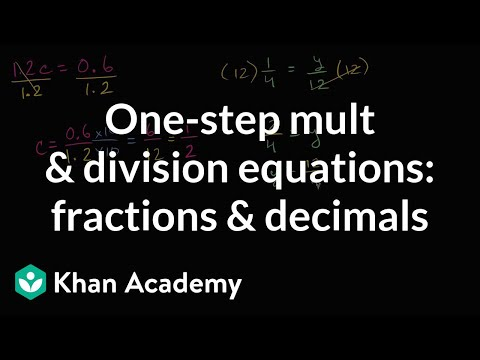By Khan Academy

Learn how to solve equations in one step by multiplying or dividing a number from both sides.ï¿½ï¿½ï¿½ï¿½ï¿½ï¿½ï¿½ï¿½ï¿½ï¿½ï¿½ï¿½These problems involve decimals and fractions.# Lattice Multiplication with Decimals

##By YourMathGal

Shows an alternate way to multiply decimals using a lattice. Youtube videos by Julie Harland are organized at http://YourMathGal.com lattice3dec1.mp4For more great math videos visit: www.youtube.com/user/videosbyjulieharland/videos . Aligned to Common Core State Standard: 5.NBT.7# Understanding moving the decimal

##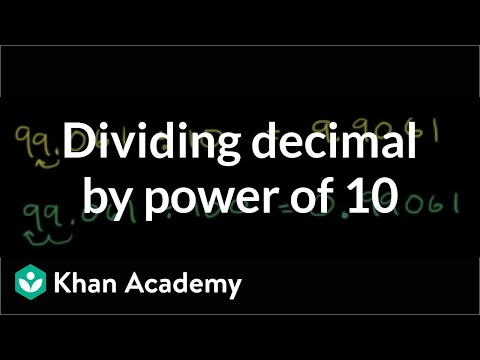By Khan Academy

When we were multiplying, we moved the decimal to the right for each power of ten. Guess what? When dividing, we move the decimal to the left for each power of ten.# Understanding moving the decimal

##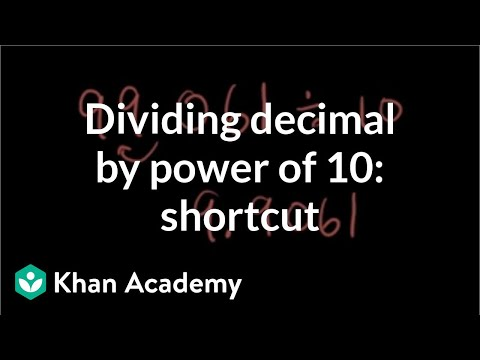By Khan Academy

Holy cow! A shortcut? Yep. For each ten you multiply or divide by, just shift that decimal to the right or left. Watch this and be amazed.# Multiplying and Dividing by Powers of 10

##By jimbabweiberg

The instructor uses an interactive white board to show that multiplying a decimal by 10 100 1 000 or 10 000 moves the decimal one two three or four places to the right. He displays a calculator to show this as well. He also explains and shows that dividing by a power of 10 moves the decimal place to the left according to the number of zeros in the number you are multiplying by.# Understanding moving the decimal

##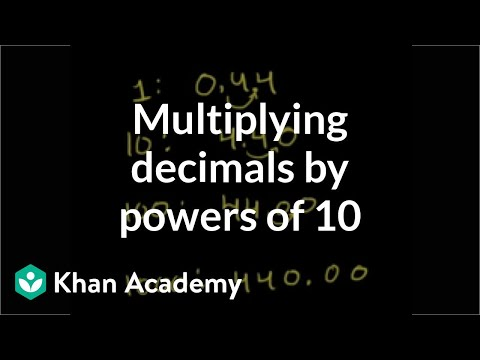By Khan Academy

You will notice in this word problem that moving the decimal to the right the same number of times as the number of zeros you multiplying by gets you the answer you desire. Check this out!